# AP SSC 10th Class Maths Solutions Chapter 14 Statistics Ex 14.2

AP State Board Syllabus AP SSC 10th Class Maths Textbook Solutions Chapter 14 Statistics Ex 14.2 Textbook Questions and Answers.

## AP State Syllabus SSC 10th Class Maths Solutions 14th Lesson Statistics Exercise 14.2

### 10th Class Maths 14th Lesson Statistics Ex 14.2 Textbook Questions and Answers

Question 1.
The following table shows the ages of the patients admitted in a hospital during a year: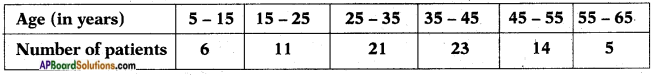Find the mode and the mean of the data given above. Compare and interpret the two measures of central tendency.
Maximum number of patients joined in the age group 35 – 45.
∴ Modal class is 35 – 45.
Lower limit of the modal class ‘l’ = 35
Class size h = 10
Frequency of modal class, f1 = 23
Frequency of the class preceding the modal class f0 = 21
Frequency of the class succeeding the modal class f2 = 14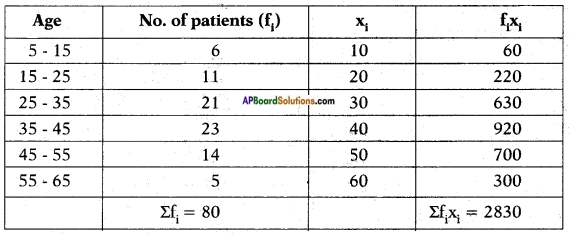∴ Mode = $$l+\frac{\left(f_{1}-f_{0}\right)}{2 f_{1}-f_{0}-f_{2}} \times h$$
$$\begin{array}{l} =35+\left(\frac{23-21}{2 \times 23-21-14}\right) \times 10 \\ =35+\left(\frac{2}{46-35}\right) \times 10 \end{array}$$
= 35 + $$\frac{2}{11}$$ × 10
= 35 + 1.81818……
= 36.8 years.
Mean x = $$\frac{\Sigma \mathrm{f}_{\mathrm{i}} \mathrm{x}_{\mathrm{i}}}{\Sigma \mathrm{f}_{\mathrm{i}}}$$
= $$\frac{2830}{80}$$
= 35.37 years.
Interpretation: Mode age is 36.8 years, Mean age = 35.37 years.
Maximum number of patients admitted in the hospital are of the age 36.8 years, while on an average the age of patients admitted to the hospital is 35.37 years. Mode is less than the Mean.Question 2.
The following data gives the information on the observed lifetimes (in hours) of 225 electrical components: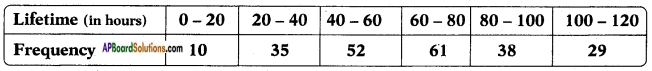Determine the modal lifetimes of the components.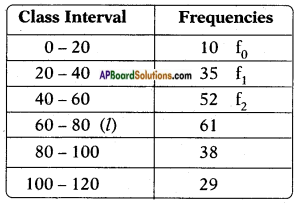Since the maximum frequency 61 is in the class 60 – 80, this is the required modal class.
Modal class frequency, f1 = 61.
Frequency of the class preceding the modal class f0 = 52
Frequency of the class succeeding the modal class f2 = 38
Lower boundary of the modal class, l = 60
Height of the class, h = 20
∴ Mode (Z) = $$l+\frac{\left(f_{1}-f_{0}\right)}{2 f_{1}-f_{0}-f_{2}} \times h$$
$$=60+\left[\frac{61-52}{2 \times 61-(52+38)}\right] \times 20$$
= 60 + $$\frac{9}{122-90}$$ × 20
= 60 + $$\frac{9}{32}$$ × 20
= 60 + 5.625
= 65.625 hours.Question 3.
The following data gives the distribution of total monthly household expenditure of 200 families of a village. Find the modal monthly expenditure of the families. Also, find the mean monthly expenditure: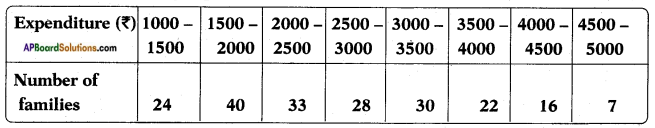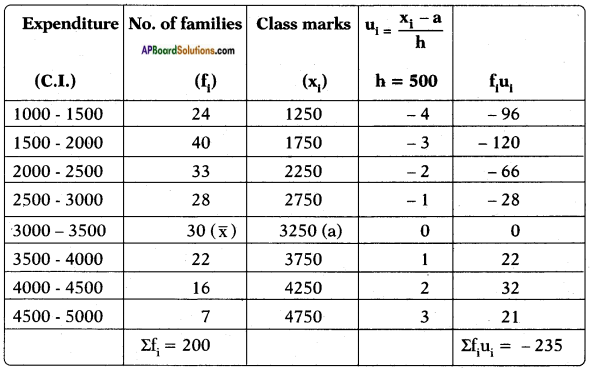Since the maximum families 40 lies in the class 1500 – 2000, this is the required modal class.
Lower boundary of the modal class (l) = 1500
Frequency of the modal class (f1) = 40
Frequency of the class preceding the modal class f0 = 24
Frequency of the class succeeding the modal class f2 = 33
Height of the class, h = 500
Hence, modal monthly income = Rs. 1847.83.
Assumed mean (a) = 3250
∑fi = 200, ∑uifi = -235
Mean monthly income = $$\overline{\mathrm{x}}=\mathrm{a}+\frac{\Sigma \mathrm{f}_{\mathrm{i}} \mathrm{u}_{\mathrm{i}}}{\Sigma \mathrm{f}_{\mathrm{i}}} \times \mathrm{h}$$
= 3250 – $$\frac{235}{200}$$ × 500
= 3250 – 587.5
= Rs. 2662.50Question 4.
The following distribution gives the state-wise, teacher-student ratio in higher secondary schools of India. Find the mode and mean of this data. Interpret the two measures.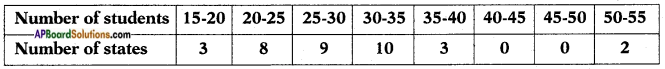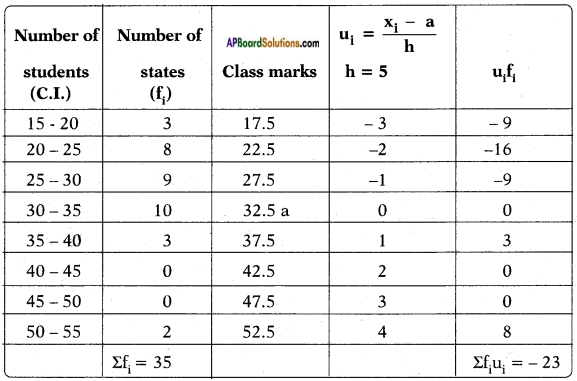Since the maximum number of states ‘10’ lies in the class interval 30-35, this is the modal class.
Lower boundary of the modal class, l = 30
Frequency of the modal class, f1 = 10
Frequency of the class preceding the modal class = f0 = 9
Frequency of the class succeeding the modal class = f2 = 3
Height of the class, h = 5
∴ Mode (Z) = $$l+\left(\frac{\mathrm{f}_{1}-\mathrm{f}_{0}}{\left(\mathrm{f}_{1}-\mathrm{f}_{0}\right)+\left(\mathrm{f}_{1}-\mathrm{f}_{2}\right)}\right) \times \mathrm{h}$$
$$=30+\frac{10-9}{(10-9)+(10-3)} \times 5$$
= 30 + $$\frac{1×5}{1+7}$$
= 30 + $$\frac{5}{8}$$
= 30 + 0.625
= 30.625
Mean $$\overline{\mathrm{x}}=\mathrm{a}+\frac{\Sigma \mathrm{f}_{\mathrm{i}} \mathrm{u}_{\mathrm{i}}}{\Sigma \mathrm{f}_{\mathrm{i}}} \times \mathrm{h}$$
a = assumed mean = 32.5; h = height of the class = 5
∴ x = 32.5 – $$\frac{23}{35}$$ × 5
= 32.5 – 3.28
= 29.22
Mean = 30.625
Mode = 29.22
Mode states have a students – teacher ratio 29.22 and on an average this ratio is 30.625.Question 5.
The given distribution shows the number of runs scored by some top batsmen of the world in one-day international cricket matches.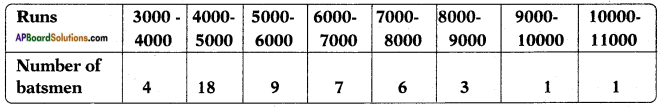Find the mode of the data.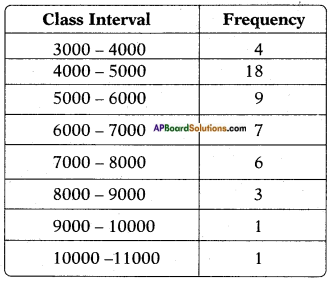Maximum number of batsmen are in the class 4000 – 5000.
∴ Modal class is 4000 – 5000.
Lower boundary of the modal class ‘l’ = 4000
Frequency of the modal class, f1 = 18
Frequency of the class preceding the modal class, f0 = 4
Frequency of the class succeeding the modal class, f2 = 9
Height of the class, h = 1000
Mode (Z) = $$l+\left(\frac{\mathrm{f}_{1}-\mathrm{f}_{0}}{\left(\mathrm{f}_{1}-\mathrm{f}_{0}\right)+\left(\mathrm{f}_{1}-\mathrm{f}_{2}\right)}\right) \times \mathrm{h}$$
Mode (Z) = $$4000+\frac{18-4}{(18-4)+(18-9)} \times 1000$$
= 4000 + $$\frac{14}{14+9}$$ × 1000
= 4000 + $$\frac{14000}{23}$$
= 4000 + 608.695
= 4608.69
≃ 4608.7 runsQuestion 6.
A student noted the number of cars passing through a spot on a road for 100 periods, each of 3 minutes, and summarised this in the table given below.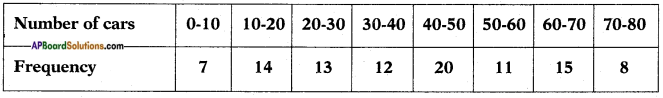Find the mode of the data.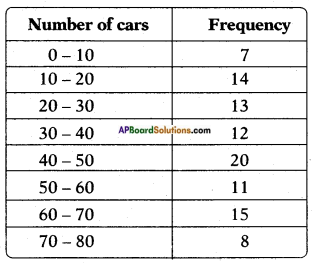Since the maximum frequency is 20, the modal class is 40 – 50.
Lower boundary of the modal class ‘l’ = 40
Frequency of the modal class, f1 = 20
Frequency of the class preceding the modal class, f0 = 12
Frequency of the class succeeding the modal class, f2 = 11
Height of the class, h = 10;
Mode (Z) = $$l+\left(\frac{\mathrm{f}_{1}-\mathrm{f}_{0}}{\left(\mathrm{f}_{1}-\mathrm{f}_{0}\right)+\left(\mathrm{f}_{1}-\mathrm{f}_{2}\right)}\right) \times \mathrm{h}$$
Mode (Z) = $$40+\frac{(20-12)}{(20-12)+(20-11)} \times 10$$
= 40 + $$\frac{8}{8+9}$$ × 10
= 40 + $$\frac{80}{17}$$
= 40 + 4.70588
= 44.705
≃ 44.7 cars